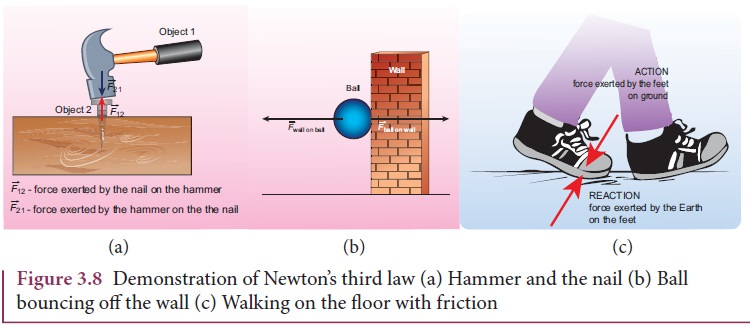Home | | Physics 11th std | Newton’s Third Law

# Newton’s Third Law

Newton’s third law assures that the forces occur as equal and opposite pairs.

Newton’s Third Law

Consider Figure 3.8(a) whenever an object 1 exerts a force on the object 2 (), then object 2 must also exert equal and opposite force on the object 1 (). These forces must lie along the line joining the two objects.Newton’s third law assures that the forces occur as equal and opposite pairs. An isolated force or a single force cannot exist in nature. Newton’s third law states that for every action there is an equal and opposite reaction. Here, action and reaction pair of forces do not act on the same body but on two different bodies. Any one of the forces can be called as an action force and the other the reaction force. Newton’s third law is valid in both inertial and non-inertial frames.These action-reaction forces are not cause and effect forces. It means that when the object 1 exerts force on the object 2, the object 2 exerts equal and opposite force on the body 1 at the same instant.

Study Material, Lecturing Notes, Assignment, Reference, Wiki description explanation, brief detail
11th Physics : UNIT 3 : Laws of Motion : Newton’s Third Law |